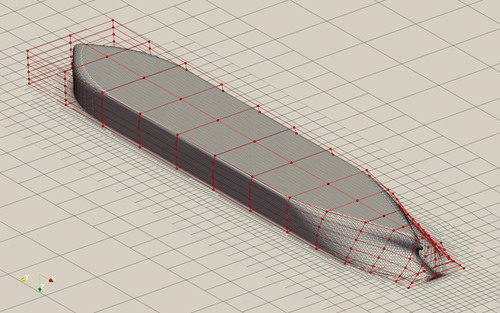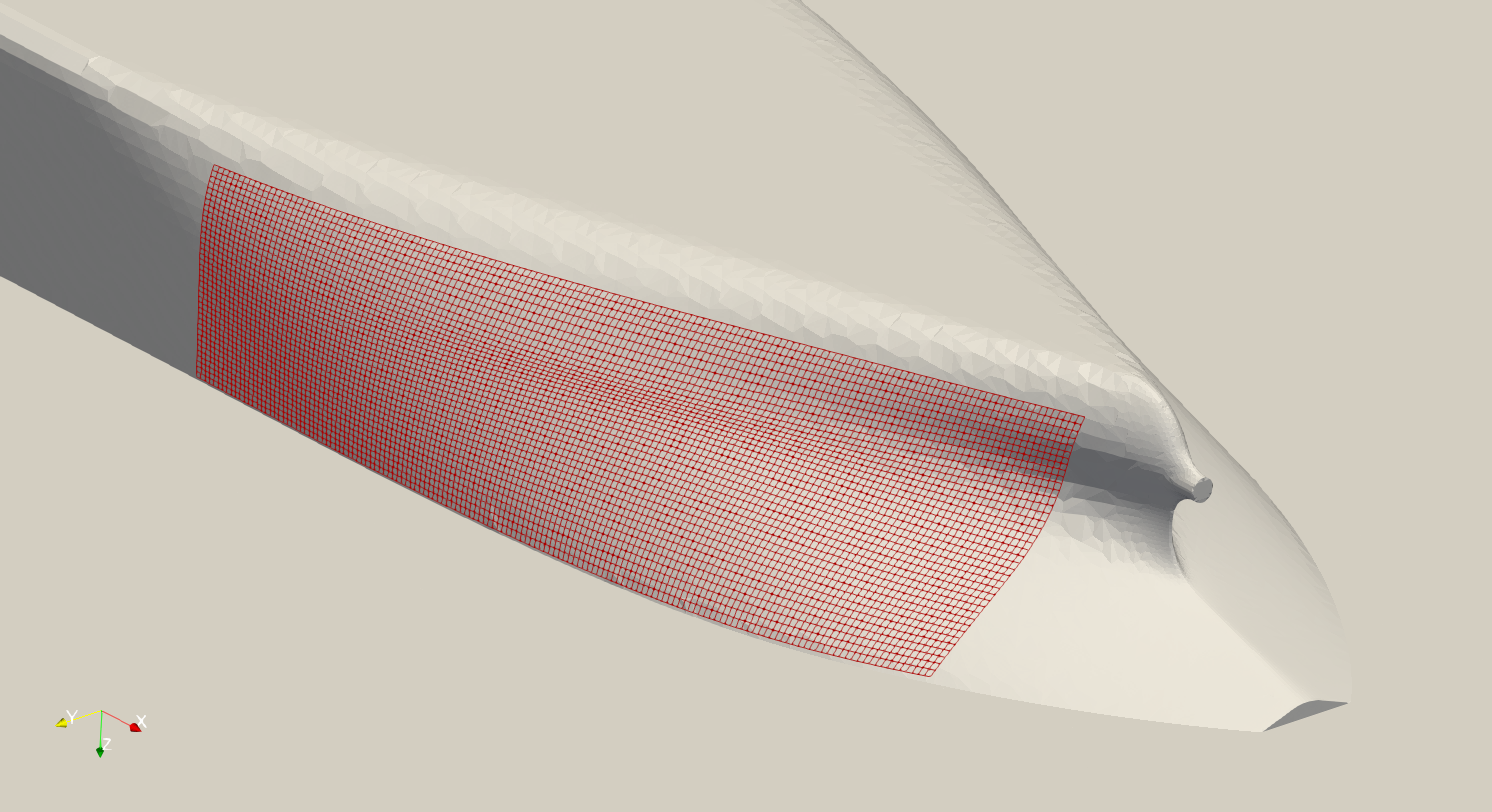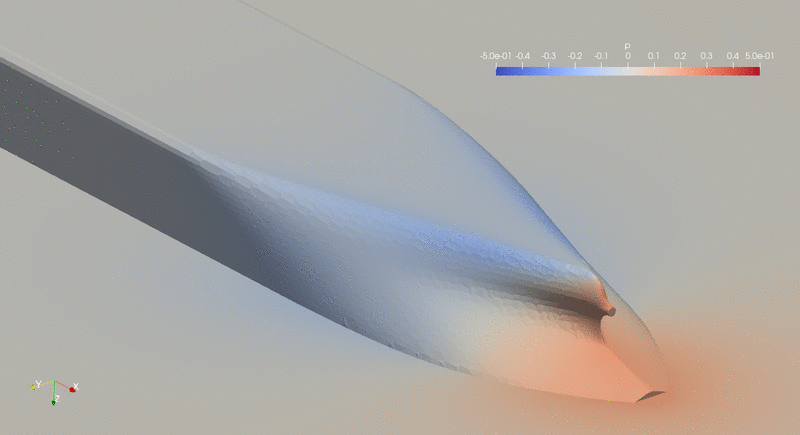The following is a hydrodynamic optimization for the JBC bulk carrier hull.

```Case: Ship hydrodynamic optimization with self-propulsion
Geometry: Japan Bulk Carrier (JBC) hull
Objective function: Drag
Design variables: 32 FFD points moving in the y direction
Constraints: Volume, thickness, symmetry, and curvature constraints (total number: 83)
Mach number: less than 0.01
Reynolds number: 7.5 million
Mesh cells: 265 K
Solver: DASimpleFoam
```Fig. 1. Mesh and FFD points for the JBC hull

To avoid wave shapes, this case impose a curvature constraint to the hull shape. The aggregated curvature (approximated max-curvature) in the optimized design is constrained to be less than 1.2 times of the curvature in the baseline design. See the following code to add the curvature constraint. Here the “hullCurv.xyz” file is a 2D surface mesh that define which area the curvature constraint is applied to. This surface mesh should be as close as possible to the hull shape. Then, pyGeo will load in this surface mesh in the FFD box and deform it along with the hull. This way, the curvature of this 2D surface mesh is the curvature of the hull.

```# Curvature constraints
"./FFD/hullCurv.xyz", curvatureType="KSmean", lower=0.0, upper=1.21, addToPyOpt=True, scaled=True
)
```Fig. 2. Example of adding curvature constraint. The red mesh is the 2D surface mesh defined hullCurv.xyz.

This case requires the IPOPT optimizer and the AD version of DAFoam. To run this case, first download tutorials and untar it. Then go to tutorials-main/JBC_Hull and run the “preProcessing.sh” script to generate the mesh:

```./preProcessing.sh
```

We recommend running this case on an HPC system with 30 CPU cores:

```mpirun -np 30 python runScript.py 2>&1 | tee logOpt.txt
```

This case ran for 40 steps. The drag reduces by 2.1%.Fig. 3. Evolution of hull shape and pressure distribution during the optimization.